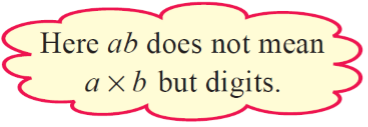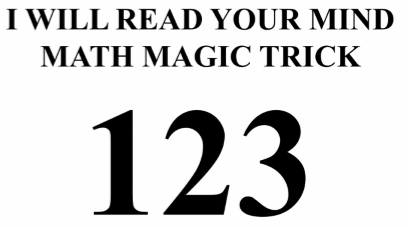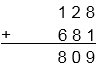×#### Thank you for registering.

One of our academic counsellors will contact you within 1 working day.

Click to Chat

1800-1023-196

+91-120-4616500

CART 0

• 0

MY CART (5)

Use Coupon: CART20 and get 20% off on all online Study Material

ITEM
DETAILS
MRP
DISCOUNT
FINAL PRICE
Total Price: Rs.

There are no items in this cart.
Continue Shopping• Complete JEE Main/Advanced Course and Test Series
• OFFERED PRICE: Rs. 15,900
• View Details

```Revision Notes on Playing with Numbers

Numbers in General Form

The general form of a number is obtained by adding the product of the digits with there place values.

1. The General Form of a Two Digit Number

ab = a × 10 + b = 10a + b

Example

93 = 10 × 9 + 3

= 90 + 3

2. The General Form of a Three Digit Number

abc = 100 × a + 10 × b + c = 100a + 10b + c

Example

256 = 100 × 2 + 10 × 5 + 6

= 200 + 50 + 6

Remark: ab doesn’t mean a × b as we generally use it like this.Games with Numbers

1. Reversing the Digits – Two Digit Number

a. The trick to divide by 11: If we have any two digit number then we will always get the remainder zero if we add the original number with its reverse number and divide it by 11. The trick behind it:

Step 1: Let us choose any two digit number ab, which can be written as (10a + b).

Step 2: Its reverse number will be ba, which can be written as (10b + a).

Step 3: Sum of both the numbers is ab + ba, we can also write as (10a + b) + (10b + a) = 11a+ 11b = 11 (a + b)

Step 4: By dividing the number by 11 we get 11 (a + b)/11 = (a + b) .

This shows that the remainder will always be zero in case of any number. The quotient is a + b, which is the sum of the digits of the given number ab.

Example

Try for the number 78.

Solution:

The given number is 78.

The reverse number is 87.

Sum of both the numbers is 78 + 87 = 165, 165 ÷ 11 = 15 and the remainder are zero.

b. The trick to divide by 9: If we have any two digit number then we will always get the remainder zero if we subtract the original number with its reverse number and divide it by 9. The trick behind it:

Step 1: Let's choose any two digit number ab, which can be written as (10a + b).

Step 2: Its reverse number will be ba, which can be written as (10b + a).

Step 3: By subtracting both the numbers i.e. ab – ba we get (10a + b) - (10b + a) = 9a - 9b = 9 (a - b)

Step 4: By dividing the number by 9 we get  9 (a - b)/9 = (a - b).

This shows that the remainder will always be zero in case of any number.

Example

Try for the number 78.

Solution:

The given number is 78.

The reverse number is 87.

Subtraction of both the numbers is 87 – 78 = 9, 9 ÷ 9 = 1 and the remainder is zero.

2. Reversing the Digits – Three Digit Number

The trick to divide by 99: If we have any three digit number then we will always get the remainder zero if we subtract the original number with its reverse number and divide it by 99. The trick behind it:

Step 1: Let's choose any three digit number abc, which can be written as (100a + 10b + c).

Step 2: Its reverse number will be cba, which can be written as (100c + 10b + a).

Step 3: By subtracting both the numbers i.e. abc - cba we get (100a + 10b + c) - (100c + 10b + a) = 99a - 99c = 99 (a - c).

Step 4: By dividing the number by 99 we get 99 (a - c)/9 = (a - c).

This shows that the remainder will always be zero in case of any number.Example

Try with the number 456

Solution:

The given number is 456.

The reverse number is 654.

Subtraction of both numbers is 654-456=198

By dividing it by 99 we get 2 i.e. 198 ÷ 99 = 2 and the remainder is zero.

3. Forming Three-digit Numbers with given three-digits.

If we have a three digit number then we will always get the remainder zero if we rearrange the number in such a way that all the three numbers are different and if we add them all and then divide it by 37. The trick behind it:

Step 1: Let us take any three digit number abc, which can be written as (100a +10b + c).

Step 2: Rearrange the number in such a way that it forms two different numbers. One number can be bca which can be written as (100b + 10c + a) and other can be cab which can be written as (100c + 10a + b).

Step 3: By adding all the three numbers i.e. abc +bca + cab we get (100a +10b + c) + (100b +10c + a) + (100c + 10a + b) = 111 (a + b + c)

Step 4: By dividing the number by 37 we will always get the remainder zero .

Example

Try for the number 397.

Solution:

Given number is 397.

By rearranging we will have two other numbers i.e. 973 and 739.

Sum of all the three numbers is 397+ 973 + 739 = 2109.

By dividing 2109 by 37 we get 57 i.e. 2109 ÷ 37 = 57 and remainder will be zero.

Letters for Digits

In this type of puzzles, the letter represents the digits and we have to crack the code. Generally, it has puzzles related to addition and multiplication.

Some important rules related to such types of puzzles:

Every letter can represent only one digit and the vice-versa.

The first digit cannot be zero Like 25 cannot be written as 025 and 0025.

Every puzzle must have only one solution.

Example

Find the value of P & Q.Solution:

Here, at the ones place the addition of P & Q is given 9. This shows that it is the sum of two single digits as the sum of two single digit numbers cannot be 19. Hence there is not any carry in this step. For the next step, 2 + P = 0 and as there was not any carry from the last step it is possible only if P = 8.
Now, 2 + 8 = 10 and 1 will be carry for the next step.
So, 1 + 1 + 6 = P. Hence, clearly, P is 8 and Q can only be 1 to satisfy the given addition.Hence, the value of P is 8 and Q is 1.1. Divisibility by 2: If the number ends with an even number i.e. 0, 2, 4, 6 and 8 then it will always be divisible by 2.

Example

Check the number 23 and 630 are divisible by 2 or not.

Solution:

23 is not divisible by 2 as its one’s digit is an odd number.

630 is divisible by 2 as its one’s digit is 0 which is even number.

2. Divisibility by 3: If the sum of all the digits of the given number is divisible by 3, then that number will also be divisible by 3.

Example

Check the number 232 and 6300 are divisible by 3 or not.

Solution:

232 is not divisible by 3 as its sum of the digits i.e. 2 + 3 + 2 = 7 is not divisible by 3.

630 is divisible by 3 as its sum of the digits i.e 6 + 3 + 0 = 9 is divisible by 3.

3. Divisibility by 4: If the last two digits of the number are divisible by 4 then that number will also be divisible by 4.​

Example

Check the number 1748 and 258 are divisible by 4 or not.

Solution:

1748 is divisible by 4 as the last two digits 48 is divisible by 4.

258 is not divisible by 4 as the last two digits 58 is not divisible by 4.

4. Divisibility by 5: If the number ends with ‘0’ or ‘5’ then it will always be divisible by 5.

Example

Check the number 23 and 630 are divisible by 5 or not.

Solution:

23 is not divisible by 5 as its one’s digit is 3.

630 is divisible by 5 as its one’s digit is 0.

5. Divisibility by 6: To be divisible by 3 a number must be divisible by 2 and 3 both. i.e. you need to check for the divisibility test of 2 and 3 with that number. Or you can say that a number must end with even number and the sum of its digit should be divisible by 3 then that number will be divisible by 6.

Example

Check the number 2341 and 6300 are divisible by 5 or not.

Solution:

2341 is not divisible by 6 as its one’s digit is odd and its sum of digit 2+3+4+1 = 10 is not divisible by 3.

6300 is divisible by 6 as its one’s digit is even and the sum of its digits 6+3+0+0=9 is divisible by 3 i.e it is divisible by both 2 and 3 hence the number is divisible by 6.

6. Divisibility by 7: To check the number is divisible with 7 or not, we need to double the last digit of the number and then subtract the result from the rest of the digits and check whether the remainder is divisible by 7 or not.If the number of digits are very large then you need to repeat the process until you get the number which could be checked for the divisibility of 7.

Example

Check the number 203 is divisible by 7 or not.

Solution:

Given number is 203

Double the last digit, 3 × 2 = 6

Subtract 6 from the remaining number 20 i.e. 20 - 6 = 14

The remainder 14 is divisible by 7 hence the number 203 is divisible by 7.

7. Divisibility by 8: If the last three digits form a number which is divisible by 8 then the whole number will be divisible by 8.

Example

Check the number 19640 is divisible by 8 or not.

Solution:

The last three digit of the number 19640 is 640.

640/8 = 80

As the number 640 is divisible by 8 hence the number 19640 is also divisible by 8.

8. Divisibility by 9: If the sum of all the digits of the given number is divisible by 9, then that number will also be divisible by 9.

Example

Check the number 232 and 6300 are divisible by 9 or not.

Solution:

232 is not divisible by 9 as its sum of the digits i.e. 2 + 3 + 2 = 7 is not divisible by 9.

630 is divisible by 9 as its sum of the digits i.e 6 + 3 + 0 = 9 is divisible by 9.

9. Divisibility by 10: If the number ends with zero then it will always be divisible by 10.

Example 1

Check the number 23 and 630 are divisible by 10 or not.

Solution:

23 is not divisible by 10 as its one’s digit is 3.

630 is divisible by 10 as its one’s digit is 0.

Example 2

If 31a5 is a multiple of 3, where a is a digit, what could be the values of a?

Solution:

As we know that the number will be divisible by 3 only if its digits sum is divisible by 3.

So let’s check the sum of its digits i.e. 3 + 1 + a + 5 = 9 + a which should be multiple of 3.

Here, a is a single digit number and also being added with 9, so any multiple of 3 can take place i.e. a can be 3 or 6 or 9 and as it is added by 9 so it could be zero also.

Hence, the value of a could be 0, 3, 6 or 9.
```### Course Features

• 728 Video Lectures
• Revision Notes
• Previous Year Papers
• Mind Map
• Study Planner
• NCERT Solutions
• Discussion Forum
• Test paper with Video Solution